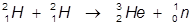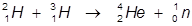# Nuclear Fusion

Nuclear fusion occurs when two elements collide and fuse into a larger element. Fusion reactions typically involve lighter elements due to the stability of the nucleus of the fused larger element (larger nuclei tend to be unstable). The resulting mass of the larger element is smaller than the combined masses of the two fusing elements. This is referred to as the mass defect.

The small amount of lost mass is converted into a huge amount of energy according to Einstein's equation, E = mc2. Due to the amount of energy created, nuclear fusion is used in hydrogen bombs and is also the power source of the sun and surrounding stars.

Examples:

1. The fusion of two hydrogen-2 atoms into a helium-3 atom.In this example, two hydrogen-2 atoms are fused into a helium-3 atom and the release of a neutron.

2. The fusion of a hydrogen-2 and hydrogen-3 atom into a helium-4 atom.In this example a hydrogen-2 and hydrogen-3 atoms fuse into a helium-4 atoms and the release of a neutron.

 Related Links: Chemistry Nuclear Chemistry Alpha Decay Beta Decay Gamma Decay Nuclear Reactions

To link to this Nuclear Fusion page, copy the following code to your site: